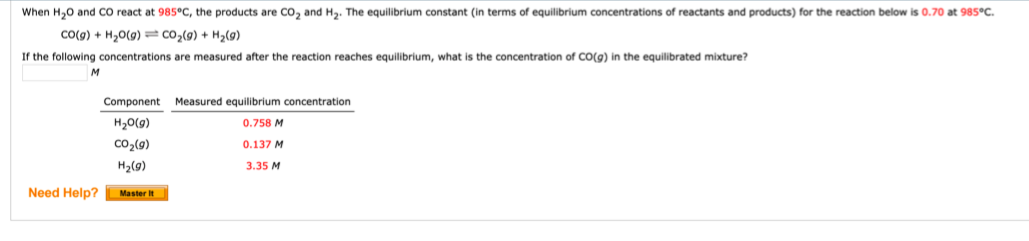# When H2O and CO react at85°C, the products are CO2 and H2. The equilibrium constant (in terms of equilibrium concentrations of reactants and products) for the reaction below is 0(at 985°C. CO(g) + H20(g) - CO2(g) + H2(g) If the following concentrations are measured after the reaction reaches equilibrium, what is the concentration of CO(g) in the equilibrated mixture? M Component Measured equilibrium concentration H20(g) 0.758 M CO2(g) 0.137 M H2(g) 3.35 M